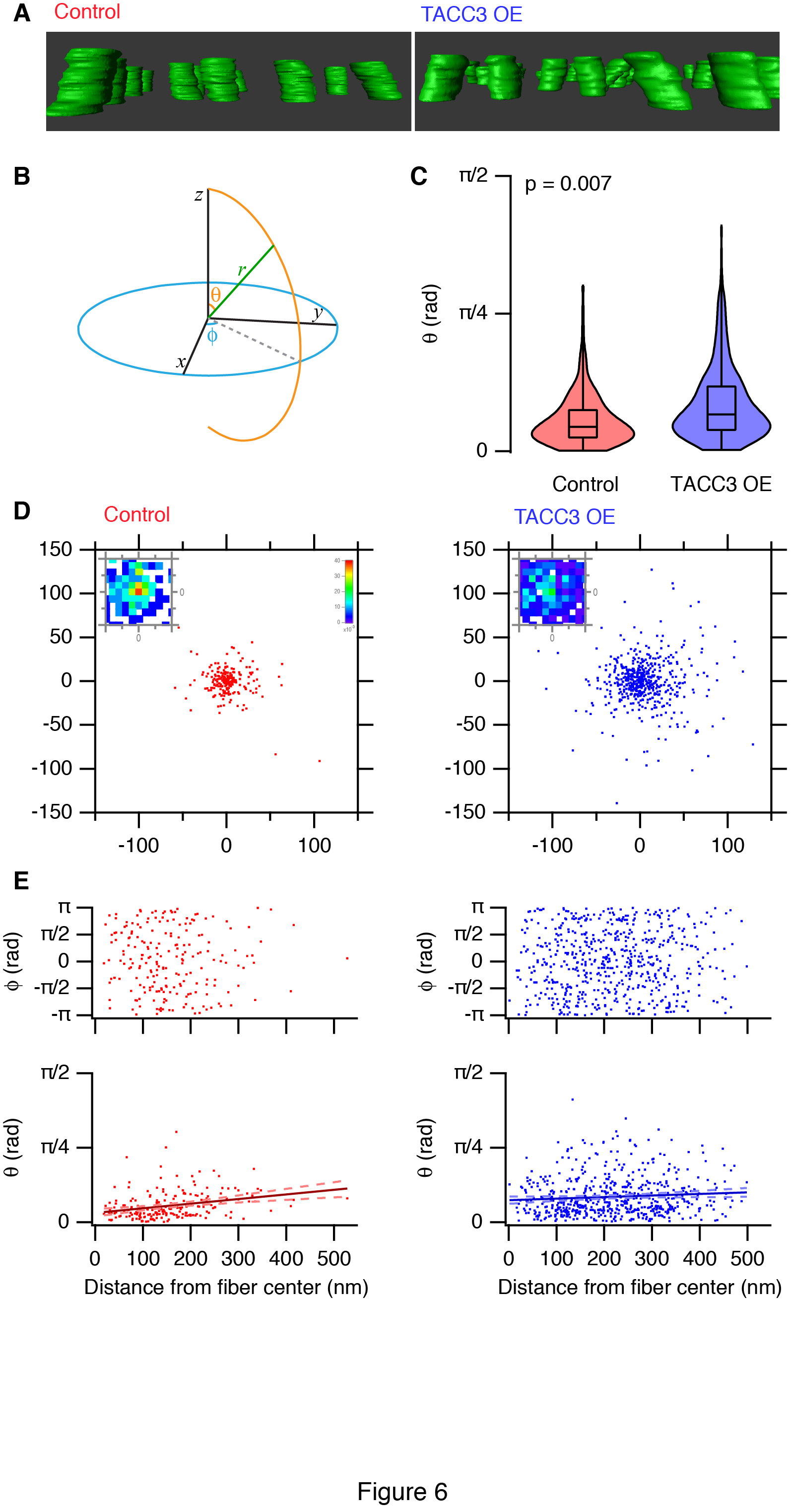# Parallel Lines: Spatial statistics of microtubules in 3D

Our recent paper on “the mesh” in kinetochore fibres (K-fibres) of the mitotic spindle was our first adventure in 3D electron microscopy. This post is about some of the new data analysis challenges that were thrown up by this study. I promised a more technical post about this paper and here it is, better late than never.In the paper we describe how over-expression of TACC3 causes the microtubules (MTs) in K-fibres to become “more wonky”. This was one of those observations that we could see by eye in the tomograms, but we needed a way to quantify it. And this meant coming up with a new spatial statistic.

After a few false starts*, we generated a method that I’ll describe here in the hope that the extra detail will be useful for other people interested in similar problems in cell biology.

The difficulty in this analysis comes from the fact that the fibres are randomly oriented, because of the way that the experiment is done. We section orthogonally to the spindle axis, but the fibre is rarely pointing exactly orthogonal to the tomogram. So the challenge is to reorient all the fibres to be able to pool numbers from across different fibres to derive any measurements. The IgorPro code to do this was made available with the paper. I have recently updated this code for a more streamlined workflow (available here).

We had two 3D point sets, one representing the position of each microtubule in the fibre at bottom of our tomogram and the other set is the position at the top. After creating individual MT waves from these point sets to work with, these waves could be plotted in 3D to have a look at them.This is done in IgorPro by using a Gizmo. Shown here is a set of MTs from one K-fibre, rotated to show how the waves look in 3D, note that the scaling in z is exaggerated compared with x and y.

We need to normalise the fibres by getting them to all point in the same direction. We found that trying to pull out the average trajectory for the fibre didn’t work so well if there were lots of wonky MTs. So we came up with the following method:

• Calculate the total cartesian distance of all MT waves in an xy view, i.e. the sum of all projections of vectors on an xy plane.
• Rotate the fibre.
• Recalculate the total distance.
• Repeat.

So we start off with this set of waves (Original). We rotate through 3D space and plot the total distance at each rotation to find the minimum, i.e. when most MTs are pointing straight at the viewer. This plot (Finding Minimum) is coloured so that hot colours are the smallest distance, it shows this calculation for a range of rotations in phi and theta. Once this minimum is found, the MT waves can be rotated by this value and the set is then normalised (you need to click on the pictures to see them properly).

Now we have all of the fibres that we imaged oriented in the same way, pointing to the zenith. This means we can look at angles relative to the z axis and derive statistics.

The next challenge was to make a measure of “wonkiness”. In other words, test how parallel the MTs are.

Violin plots of theta don’t really get across the wonkiness of the TACC3 overexpressed K-fibres (see figure above). To visualise this more clearly, each MT was turned into a vector starting at the origin and the point where the vector intersected with an xy plane set at an arbitrary distance in z (100 nm) was calculated. The scatter of these intersections demonstrates nicely how parallel the MTs are. If all MTs were perfectly parallel, they would all intersect at 0,0. In the control this is more-or-less true, with a bit of noise. In contrast, the TACC3-overexpressed group have much more scatter. What was nice is that the radial scatter was homogeneous, which showed that there was no bias in the acquisition of tomograms. The final touch was to generate a bivariate histogram which shows the scatter around 0,0 but it is normalised for the total number of points. Note that none of this possible without the first normalisation step.

Parallelism

The only thing that we didn’t have was a good term to describe what we were studying. “Wonkiness” didn’t sound very scientific and “parallelness” was also a bit strange. Parallelism is a word used in the humanities to describe analogies in art, film etc. However, it seemed the best term to describe the study of how parallel the MTs in a fibre are.

With a little help from my friends

The development of this method was borne out of discussions with Tom Honnor and Julia Brettschneider in the Statistics department in Warwick. The idea for the intersecting scatter plot came from Anne Straube in the office next door to me. They are all acknowledged in our paper for their input. A.G. at WaveMetrics helped me speed up my code by using MatrixOP and Euler’s rotation. His other suggestion of using PCA to do this would undoubtedly be faster, but I haven’t implemented this – yet. The bivariate histograms were made using JointHistogram() found here. JointHistogram now ships with Igor 7.

* as we describe in the paper

Several other strategies were explored to analyze deviations in trajectory versus the fiber axis. These were: examining the variance in trajectory angles, pairwise comparison of all MTs in the bundle, comparison to a reference MT that represented the fiber axis, using spherical rotation and rotating by an average value. These produced similar results, however, the one described here was the most robust and represents our best method for this kind of spatial statistical analysis.

The post title is taken from the Blondie LP “Parallel Lines”.